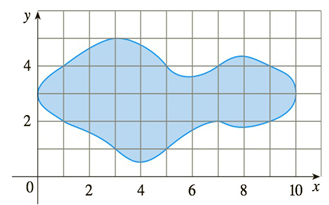Chapter 5.3, Problem 28E

Chapter
Section
Textbook Problem

# If the region shown in the figure is rotated about the y-axis to form a solid, use the Midpoint Rule with n = 5 to estimate the volume of the solid.To determine

To estimate:

The volume of solid using the midpoint rule.

Explanation

1) Concept:

i. If x  is the radius of the typical shell, then the circumference =2πx and the height is y=f(x)

ii. By the shell method, the volume of the solid by rotating the region under the curve y=f(x) about x-axis from a to b is

V= ab2πx f(x)dx

where  0a<b

iii. To approximate the integral is same as finding the Riemann sum for the midpoints. Use the formula and find the value of the integral.

2) Formula:

The Riemann sum for the midpoints:

Mn=i=1ng(xi-)x~abg(x)dx

where, xi-=12xi-1+xi and  x=b-an, n is the number of the subintervals.

3) Given:

n=5

4) Calculation:

The volume of the solid is

V= ab2πx f(x)dx

Assume that, gx=2πx fx

So, volume of solid is

V= abg(x)dx

where x is the radius and fx is the height of each cylinder.

So, fx=yi-yi-1

where yi is the upper part of the region and yi-1 is the lower part of the region

From the given figure,

The region is bounded between 0 to 10

That means a=0, b=10 and n=5

So the subintervals are 0, 2, 2, 4,

### Still sussing out bartleby?

Check out a sample textbook solution.

See a sample solution

#### The Solution to Your Study Problems

Bartleby provides explanations to thousands of textbook problems written by our experts, many with advanced degrees!

Get Started

#### Convert the expressions in Exercises 8596 radical form. y7/4

Finite Mathematics and Applied Calculus (MindTap Course List)

#### 27r63s2t4

Applied Calculus for the Managerial, Life, and Social Sciences: A Brief Approach

#### Given the Taylor Series , a Taylor series for ex/2 is:

Study Guide for Stewart's Multivariable Calculus, 8th

#### The solution to y = y2 with y(1)=13 is: a) y=1x+2 b) y=lnx+13 c) y=12x216 d) y=12x16

Study Guide for Stewart's Single Variable Calculus: Early Transcendentals, 8th